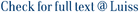We study the optimal control of path-dependent McKean–Vlasov equations valued in Hilbert spaces motivated by non-Markovian mean-field models driven by stochastic PDEs. We first establish the well-posedness of the state equation, and then we prove the dynamic programming principle (DPP) in such a general framework. The crucial law invariance property of the value function V is rigorously obtained, which means that V can be viewed as a function on the Wasserstein space of probability measures on the set of continuous functions valued in Hilbert space. We then define a notion of pathwise measure derivative, which extends the Wasserstein derivative due to Lions (Lions (Audio Conference, 2006–2012)), and prove a related functional Itô formula in the spirit of Dupire ((2009), Functional Itô Calculus, Bloomberg Portfolio Research Paper No. 2009-04-FRONTIERS) and Wu and Zhang (Ann. Appl. Probab. 30 (2020) 936–986). The Master Bellman equation is derived from the DPP by means of a suitable notion of viscosity solution. We provide different formulations and simplifications of such a Bellman equation notably in the special case when there is no dependence on the law of the control.

Optimal control of Path-dependent Mckean–Vlasov SDEs in infinite-dimension / Cosso, A.; Gozzi, Fausto; Kharroubi, I.; Pham, H.; Rosestolato, Mauro. - In: THE ANNALS OF APPLIED PROBABILITY. - ISSN 1050-5164. - 33:4(2023), pp. 2863-2918. [10.1214/22-AAP1880]

### Optimal control of Path-dependent Mckean–Vlasov SDEs in infinite-dimension

#### Abstract

We study the optimal control of path-dependent McKean–Vlasov equations valued in Hilbert spaces motivated by non-Markovian mean-field models driven by stochastic PDEs. We first establish the well-posedness of the state equation, and then we prove the dynamic programming principle (DPP) in such a general framework. The crucial law invariance property of the value function V is rigorously obtained, which means that V can be viewed as a function on the Wasserstein space of probability measures on the set of continuous functions valued in Hilbert space. We then define a notion of pathwise measure derivative, which extends the Wasserstein derivative due to Lions (Lions (Audio Conference, 2006–2012)), and prove a related functional Itô formula in the spirit of Dupire ((2009), Functional Itô Calculus, Bloomberg Portfolio Research Paper No. 2009-04-FRONTIERS) and Wu and Zhang (Ann. Appl. Probab. 30 (2020) 936–986). The Master Bellman equation is derived from the DPP by means of a suitable notion of viscosity solution. We provide different formulations and simplifications of such a Bellman equation notably in the special case when there is no dependence on the law of the control.
##### Scheda breve Scheda completa Scheda completa (DC)2023
dynamic programming principle, functional Itô calculus, Master Bellman equation, Path-dependent McKean–Vlasov SDEs in Hilbert space, pathwise measure derivative, viscosity solutions
Optimal control of Path-dependent Mckean–Vlasov SDEs in infinite-dimension / Cosso, A.; Gozzi, Fausto; Kharroubi, I.; Pham, H.; Rosestolato, Mauro. - In: THE ANNALS OF APPLIED PROBABILITY. - ISSN 1050-5164. - 33:4(2023), pp. 2863-2918. [10.1214/22-AAP1880]
File in questo prodotto:
File
22-AAP1880.pdf

Solo gestori archivio

Tipologia: Versione dell'editore
Licenza: Tutti i diritti riservati
Dimensione 647.26 kB
Utilizza questo identificativo per citare o creare un link a questo documento: `https://hdl.handle.net/11385/233799`
•2
•3